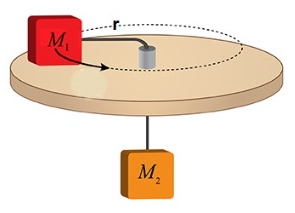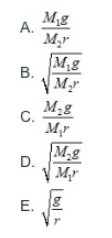# Problem: Two blocks, which can be modeled as point masses, are connected by a massless string which passes through a hole in a frictionless table. A tube extends out of the hole in the table so that the portion of the string between the hole and My remains parallel to the top of the table. If block M2's acceleration is zero (in static equilibrium), what is a correct expression for the angular speed ω of block M1, in terms of the masses, radius of the circle r, and g?

###### FREE Expert Solution

Centripetal force:

$\overline{){\mathbf{F}}{\mathbf{=}}{\mathbf{m}}{{\mathbf{a}}}_{{\mathbf{c}}}{\mathbf{=}}{\mathbf{m}}{\mathbf{r}}{{\mathbf{\omega }}}^{{\mathbf{2}}}}$

Let's determine the tension in the string due to M1:

87% (48 ratings)###### Problem Details

Two blocks, which can be modeled as point masses, are connected by a massless string which passes through a hole in a frictionless table. A tube extends out of the hole in the table so that the portion of the string between the hole and My remains parallel to the top of the table. If block M2's acceleration is zero (in static equilibrium), what is a correct expression for the angular speed ω of block M1, in terms of the masses, radius of the circle r, and g?